# Class 11 Chemistry Chapter 1 – Some Basic Concepts of Chemistry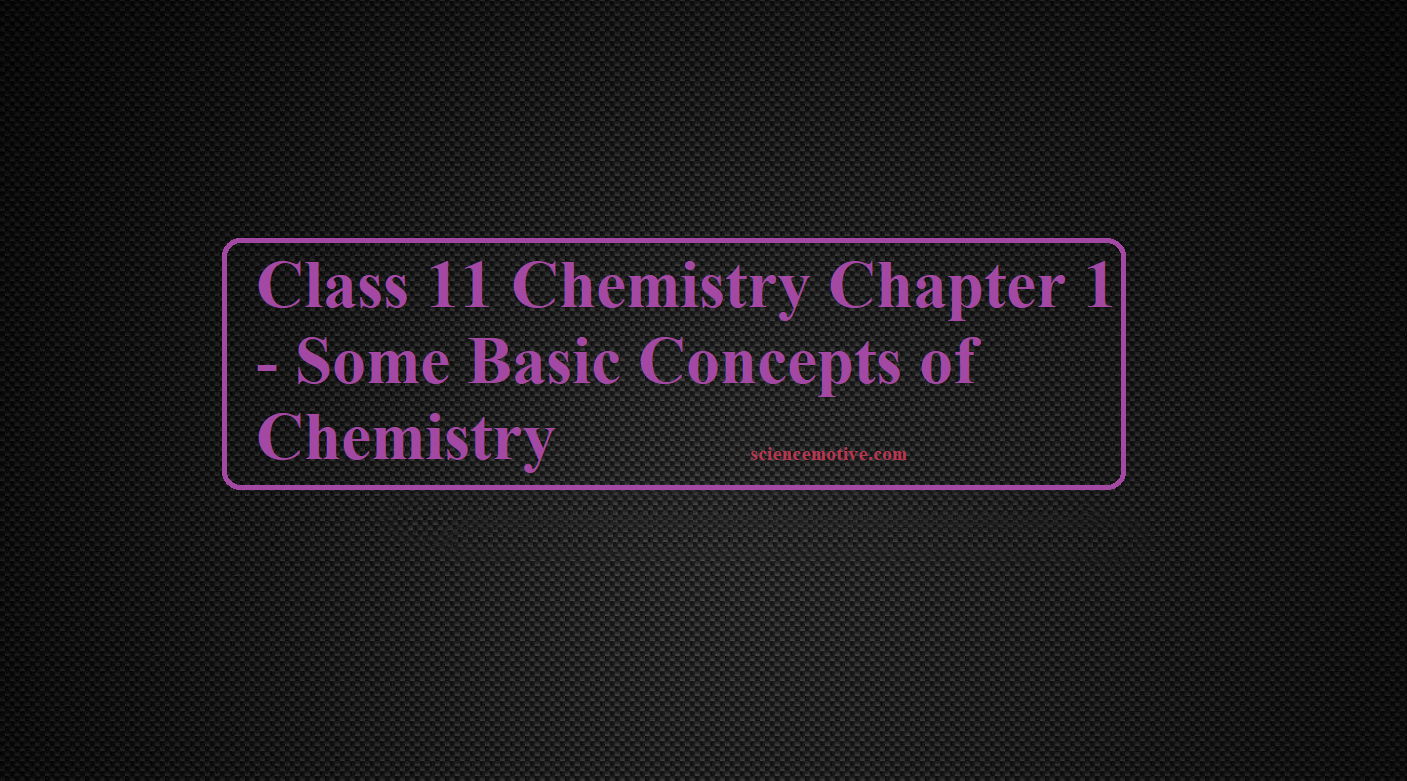# Class 11 Chemistry Chapter 1 – Some Basic Concepts of Chemistry

Sub Topics for the Post Class 11 Chemistry Chapter 1 – Some Basic Concepts of Chemistry

Solved worksheet Some Basic Concepts of Chemistry

Solved Questions Basic Concepts of Chemistry

Important Solved Questions on Mole Concept

Chemistry is the branch of science that deals with the properties, structure, and composition of matter. There are a large number of branches for Chemistry. Some of them are:
Inorganic Chemistry                           Organic Chemistry
Physical Chemistry                            Analytical Chemistry
Polymer Chemistry                            Biochemistry
Medicinal Chemistry                          Industrial Chemistry
Hydrochemistry                                  Electrochemistry
Green Chemistry

Matter: Matter is anything that occupies space, has a definite mass, and can be perceived by any of our sense organs. Based on the physical state we can divide matter into different categories.
Solid-State                                          Liquid State
Gaseous State                                    Plasma State
Bose-Einstein Condensate             Fermionic condensate

Classification of Matter: On the basis of Matter composition it can be divided into two categories:

• Pure Substances
• Mixtures

Pure substances are made up of the same type of particles. E.g. sodium (Na), Potassium (K), Hydrogen (H), Oxygen (O), Helium (He), Carbon dioxide (CO2), water (H2O), ammonia (NH3), cane sugar (C12H22O11), etc.
These are of two types:

• Elements
• Compounds

Elements are pure substances that contain only one type of particle. These particles may be atoms or molecules. For eg: Hydrogen, Nitrogen, Sodium, Potassium, Lithium, Calcium, Phosphorus, etc.

Compounds are pure substances that contain more than one type of atoms. E.g. CO2, H2O, NH3, H2SO4, etc.

Mixtures are made up of two or more types of particles. E.g. all types of solutions, gold ornaments, seawater, muddy water, air, etc.
There are two types of mixtures:

• Homogeneous Mixture
• Heterogeneous Mixture

Homogeneous Mixture: Mixture with uniform distribution of particles or mixture with no visible (separation) boundaries of particles. For eg. all types of solutions, water in milk, ink in water, air, etc.

Heterogeneous Mixture: Mixture with no uniform distribution of particles or mixture with visible separation boundaries. For eg. Salt in water, oil in water, soil, etc.

Mass: Mass of a substance is the amount of matter present in it. Its SI unit is the kilogram (kg).

Weight: Weight is the force exerted by gravity on an object. Its SI unit is the newton (N).

• The mass of a substance is constant whereas its weight may vary from one place to another due to change in gravity.

Volume (V): It is the space occupied by a body. Its SI unit is m3 (cubic meter). Other units are cm3 (cubic centimeter), mL, L, etc.
1 m3 = 106cm3                 1 cm3 = 1 mL
1 L = 103 cm3                         1 dm3 = 103 cm3
Density (d): Density of a substance is its amount of mass present in unit volume.
Density = mass/volume.
Its SI unit is kg/m3.
It is commonly expressed in g/cm3.
Temperature: It is the degree of hotness or coldness of a body. It is commonly expressed in degrees Celsius (0C). Other units are degrees Fahrenheit (0F), Kelvin (K), etc. its SI unit is Kelvin (K).
Degree Celsius and degree Fahrenheit are related as:
0F = 9/5(0C) + 32
Degree Celsius and Kelvin are related as:
K = 0C + 273.15
Standard Temperature Pressure (STP): 0 0C (273.15 K) temperature and 1 atm pressure.
Normal Temperature Pressure (NTP): 20 0C (293.15 K) temperature and 1 atm pressure. Standard Ambient Temperature Pressure (SATP): 25 0C (298.15 K) temperature and 1 atm pressure.
The SI system has seven base units which pertain to a few fundamental scientific quantities

 Base Physical Quantity Symbol for quantity Name of SI units The symbol for SI units Length l Metre m Mass m kilogram kg Time t Second s Electric current I Ampere A Thermodynamic temperature T Kelvin k Amount of substance n Mole mol

Class 11 Chemistry Chapter 1

Precision: It indicates how closely repeated measurements match each other.

Accuracy: It indicates how closely a measurement matches the correct or expected value.

Scientiﬁc Notation: Any number can be represented in the form where n is an exponent having positive or negative values and N can vary between 1 to 10. Expressing a number in form N × 10n, and N can vary between 1 to 10.

Signiﬁcant ﬁgures: These are meaningful digits that are known with certainty. The uncertainty is indicated by writing the certain digits and the last uncertain digit. Thus, if we write a result as 11.2 mL, we say the 11 is certain and 2 is uncertain and the uncertainty would be 1 in the last digit.
There are certain rules for determining the number of significant figures. These are:

1. All non-zero digits are significant. For example, in 285 cm, there are three significant figures and in 0.25 mL, there are two significant figures.
2. Zeros preceding to first non-zero digit are not significant. Such zero indicates the position of the decimal point. Thus, 0.03 has one significant figure and 0.0052 has two significant figures.
3. Zeros between two non-zero digits are significant. Thus, 2.005 has four significant figures.
4. Zeros at the end or right of a number are significant if they are on the right side of the decimal point; otherwise, they are not significant. For example, 0.200 g has three significant figures.
5. Exact numbers have an infinite number of significant figures. For example, in 2 balls or 20 eggs, there are infinite significant figures since these are exact numbers and can be represented by writing an infinite number of zeros after placing a decimal i.e., 2 = 2.000000 or 20 = 20.000000

When numbers are written in scientific notation, the number of digits between 1 and 10 gives the number of significant figures. For e.g. 4.01×102 has three significant figures, and 8.256 × 10–3 has four significant figures.

Class 11 Chemistry Chapter 1

Laws of Chemical Combinations:

Law of Conservation of Mass: Antoine Lavoisier established the Law of Conservation of Mass. It states that matter can neither be created nor destroyed.
In other words, we can say that during any physical or chemical change, the total mass of reactants is equal to the total mass of products. For eg.
Consider the reaction 2H2 + O2 → 2H2O
Here 4 g of H2 combines with 32 g of O2 to form 36 g of water.
Total mass of reactants = 4 + 32 = 36g. The total mass of products = 36 g

Law of Deﬁnite Proportions: A given compound always contains the same elements in the same proportion by mass.
For Example, Carbon dioxide can be formed in the atmosphere by various methods like respiration, burning of fuels, the reaction of metal carbonates and bicarbonates with acid, etc. All these samples of CO2 contain only two elements Carbon and Oxygen combined in a mass ratio of 3:8.

Law of Multiple Proportions: When two elements combine to form two or more compounds, then the different masses of one element, which combine with a ﬁxed mass of the other, bear a simple ratio to one another.
Hydrogen combines with oxygen to form two compounds – water and hydrogen peroxide.

Hydrogen + Oxygen → Water
2g            16g          18g
Hydrogen + Oxygen → Hydrogen Peroxide
2g               32g                34g
Here, the masses of oxygen (i.e. 16 g and 32 g) which combine with a fixed mass of hydrogen (2g) bear a simple ratio, i.e. 16:32 or 1: 2.

Gay Lussac’s Law: Under similar conditions of temperature and pressure whenever gases combine together, they do so in terms of volume.
e.g.,  2 H2 (g)  +  O2 (g)  →  2 H2O (g)
2 vol          1 vol           2 Vol     (at same T, P)

Avogadro’s Law: Under similar conditions of temperature and pressure equal volume of all gases contains an equal number of molecules.
For example: If we take 10L each of NH3, N2, O2, and CO2 at the same temperature and pressure, all of them contain the same number of moles and molecules.

Dalton’s atomic theory: In 1808, Dalton published ‘A New System of Chemical Philosophy’ in which he proposed the following:
(i) Matter consists of indivisible atoms.
(ii) All the atoms of a given element have identical properties including identical mass. Atoms of different elements differ in mass.
(iii) Compounds are formed when atoms of different elements combine in a fixed ratio.
(iv) Chemical reactions involve the reorganization of atoms. These are neither created nor destroyed in a chemical reaction.

Class 11 Chemistry Chapter 1

Atoms and Molecules: Atom is the smallest particle of an element. Molecules are the smallest particle of a substance. A molecule has all the properties of that substance.  Types of molecules Based on the type of atoms, there are two types of molecules – The homonuclear molecule and the Heteronuclear molecule. A molecule containing only one type of atom is called a Homonuclear molecule. e.g. H2, O2, N2, O3 (ozone), etc Heteronuclear molecules contain different types of atoms. E.g. CO2, H2O, C6H12O6, NH3, etc. Based on the no. of atoms there are three types of molecules – monoatomic, diatomic, and polyatomic molecules.

• Monoatomic molecules contain only one atom. E.g. all metals, noble gases like He, Ne, Ar, etc.
• Diatomic molecules contain 2 atoms. E.g. H2, O2, N2, halogens (F2, Cl2, Br2, and I2).
• Polyatomic molecules contain more than two atoms. E.g. ozone (O3), Phosphorus (P4), Sulphur (S8), etc.

Atomic Mass: It is deﬁned as the average relative mass of an atom of an element as compared to the mass of an atom of carbon 12 taken as 12.  Atomic mass is represented by u (uniﬁed mass).
Atomic mass unit (amu): 1/12th the mass of a C12 atom is called the atomic mass unit (amu).  i.e. 1 amu = 1/12 × mass of a C12 atom = 1.66 × 10-24 g = 1.66 ×10-27 kg
Today, ‘amu’ has been replaced by ‘u’ which is known as unified mass.
Gram Atomic Mass: It may be defined as the mass of 1-mole atoms of an element. For eg. Mass of one oxygen atom = 16 amu = 16/NA gms
Mass of NA Oxygen atom = 16/NA×NA = 16 gms
Average atomic mass: All most all the elements have isotopes. So, we can calculate an average atomic mass of an element by considering the atomic mass of the isotopes and their relative abundance. For e.g. Chlorine has two isotopes 35Cl and 37Cl in the ratio 3:1. So the average atomic mass Cl = (3×35 + 1×37)/4 = 35.5

Molecular mass: It is the sum of the atomic mass of the elements present in the molecule. For example: Molecular mass of CH4 = (1 × 12) + (4 × 1) = 16 u
Molecular mass of H2SO4 is calculated as: 2 × 1 + 32 + 4 × 16 = 98 u.
Mole (n): It is the amount of a substance that contains as many particles or entities as the number of atoms in exactly 12 grams of pure C-12.
1 mole of any substance contains 6.022 x 1023 atoms. This number is known as the Avogadro number or Avogadro constant (NA or N0).
1 mole of a substance = Molar mass of substance = Avogadro’s Number of chemical units = 22.4L volume at STP of a gaseous substance.
e.g., 1 mole of CH4 = 16g of CH4 = 6.022 × 1023 molecules of CH4 = 22.4L at STP.
n =   Given Mass
Molecular Mass
1 mol of hydrogen atoms = 6.022×1023 atoms
1 mol of water molecules = 6.022×1023 water molecules
1 mol of sodium chloride = 6.022 × 1023 formula units of sodium chloride

Molar volume: It is the volume of 1 mole of any substance. At standard temperature and pressure (STP), the molar volume of any gas = 22.4 L (or, 22400 mL). i.e. 22.4 L of any gas at STP contains 1 mole of the gas or 6.022 x 1023 molecules of the gas and its mass = molar mass. For e.g. 22.4 L of hydrogen gas = 1 mole of H2 = 6.022×1023 molecules of hydrogen = 2 g of H2

1 Mole of atoms = Gram atomic mass or molar mass = 6.022 x 1023 molecules = 22.4 L at NTP (00C, 1atm) = 22.7 L at STP (00C, 1 bar).

Rules in Brief: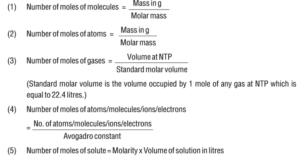Class 11 Chemistry Chapter 1

Percentage composition: It is the percentage of each element present in 100g of a substance. Percentage composition (mass percent) of an element

= Mass of that element in the compound × 100
Molar mass of the compound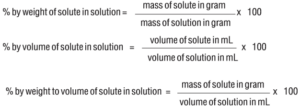Empirical Formula: It represents the simplest whole-number ratio of various atoms present in a compound. e.g., CH is the empirical formula of benzene (C6H6).

Molecular Formula: It shows the exact number of different atoms present in a molecule of a compound. e.g., C6H6 is the molecular formula of benzene.

Relationship between empirical and molecular formulae:
Molecular formula = n × Empirical formula
Where; n = Molecular Mass
Empirical Formula Mass

Following Steps Should be Followed to Determine the Empirical Formula of the Compound:
Step 1: Conversion of mass percent of various elements into grams.
Step 2: Convert mass obtained into the number of moles.
Step 3: Divide the mole value obtained by the smallest mole value (out of the mole value of various elements calculated)
Step 4: If the ratios are not whole numbers, then they may be converted into whole numbers by multiplying with the suitable coefficient.
Step 5: Write the empirical formula by mentioning the numbers after writing the symbols of the respective elements. For Example:

Class 11 Chemistry Chapter 1

Que. A compound on analysis gave the following percentage composition Na=14.31% S=9.97% H=6.22% O=69.5%. Calculate the molecular formula of the compound on the assumption that all hydrogen in the compound is present in the combination with oxygen as the water of crystallization. The molecular mass of the compound is 322. Na=23 S=32 O=16 H=1.
Ans: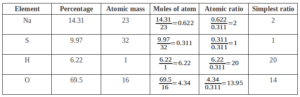Empirical Formula = Na2SH20O14
We Know
Molecular formula = n × Empirical formula
Where; n = Molecular Mass
Empirical Formula Mass
n = 322 = 1
322
Molecular Formula = 1 × Na2SH20O20 = Na2SH20O20
Final Molecular Formula – Na2SO4.10H2O

Limiting reagent (Limiting reactant): The reagent which limits a reaction or the reagent which is completely consumed in a chemical reaction is called limiting reagent or limiting reactant. For e.g. in the reaction
2SO2(g) + O2(g)    2 SO3(g), 2 moles of SO2 reacts completely with 1 mole of O2 to form 2 moles of SO3. If we take 10 moles each of SO2 and O2, we get only 10 moles of SO3 because 10 moles of SO2 require only 5 moles of O2 for the complete reaction. So here SO2 is the limiting reagent and 5 moles of O2 remain unreacted.

Reactions in solutions:
Solutions are homogeneous mixtures containing 2 or more components. The component which is present in a larger quantities is called Solvent and the other components are called Solutes. Or, the substance which is dissolved is called the solute, and the substance in which solute is dissolved is called the solvent. For e.g. in NaCl solution, NaCl is the solute and water is the solvent. A solution containing only 2 components is called Binary Solution. If the solvent is water, it is called an aqueous solution.

Concentration Can be Expressed in the Following Ways:
Mass percent (w/w or m/m): It is defined as the number of parts solute present in 100 parts by mass of solution. i.e.
Mass % of a component =   Mass of solute  × 100
Mass of solution

Molarity (M): It is defined as the number of moles of solute dissolved per liter of solution. i.e.

Molarity (M) = Number of moles of solute (n)
Volume of the solution in litre (V)
1 M NaOH solution means 1 mole of NaOH is present in 1 L of solution.
Molarity equation:    M1V1   =    M2V2
(Before dilution) = (After Dilution)
The molarity of a solution decreases on increasing temperature. The molarity of pure water is 55.56 mol L-1.

Molality (m) = It is the number of moles of solute dissolved per 1000g (1kg) of solvent.
Molality =  Number of moles of solute
Mass of solvent in kg

Among the above concentration terms, Molarity depends on temperature because it is related to volume, which changes with temperature. Whereas Molality is Independent of Temperature.
Mole Fraction (x): It is defined as the ratio of the number of moles of a particular component to the total number of moles of solution. i.e.
Mole fraction of a component =  Number of moles of the component
Total number of moles of all the components
Let us consider we have two components 1 and 2. Therefore mole fraction of component 1 is x1, and component 2 is x2.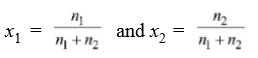x1 + x2 = 1 i.e the sum of the mole fractions of all the components in a solution is always equal to 1.

Class 11 Chemistry Chapter 1